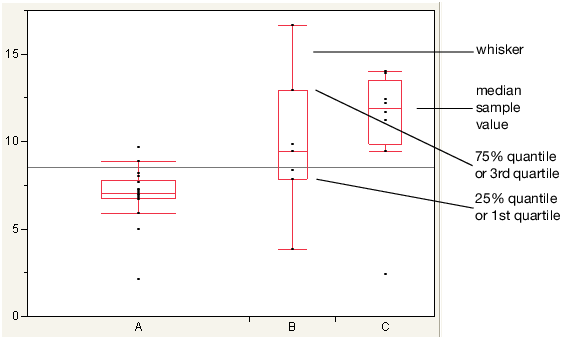Basic Analysis > Oneway Analysis > Quantiles > Outlier Box Plots
Publication date: 08/13/2020

## Outlier Box Plots

The outlier box plot is a graphical summary of the distribution of data. Note the following aspects about outlier box plots (Figure 6.7):

The horizontal line within the box represents the median sample value.

The ends of the box represent the 75th and 25th quantiles, also expressed as the 3rd and 1st quartile, respectively.

The difference between the 1st and 3rd quartiles is called the interquartile range.

Each box has lines, sometimes called whiskers, that extend from each end. The whiskers extend from the ends of the box to the outermost data point that falls within the distances computed as follows:

3rd quartile + 1.5*(interquartile range)

1st quartile - 1.5*(interquartile range)

If the data points do not reach the computed ranges, then the whiskers are determined by the upper and lower data point values (not including outliers).

Figure 6.7 Examples of Outlier Box Plots